# SSAT Upper Level Math : Tangent Lines

## Example Questions

### Example Question #1 : How To Find The Slope Of A Tangent Line

There is a circle on a coordinate plain. Its perimeter passes through the point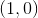. At this point meets a tangent line, which also passes through the point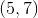. What is the slope of the line perpindicular to this tangent line?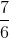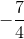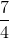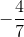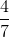Explanation:

In this kind of problem, it's important to keep track of information given about your line of interest. In this case, the coordinates given set up the stage for us to be able to get to our line of focus - the line perpendicular to the tangent line. In order to determine the perpendicular line's slope, the tangent line's slope must be calculated. Keeping in mind that: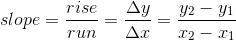where y2,x2 and y1,x1 are assigned arbitrarily as long as the order of assignment is maintained.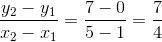which is the slope of the tangent line.

To calculate the perpendicular line, we have to remember that the product of the tangent slope and the perpendicular slope will equal -1.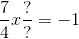, the perpendicular slope can then be calculated as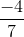### Example Question #1 : How To Find The Slope Of Tangent Lines

Find the slope of a tangent line at point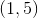if the equation is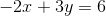.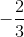Explanation:

Rewrite the linear equation in standard form to slope-intercept form,.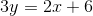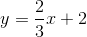Since the slope of every point of this line is, the slope of the tangent line at the given point should also be.

### Example Question #461 : Ssat Upper Level Quantitative (Math)

Find the slope of a tangent line at point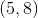if the equation of the function is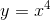.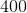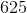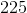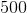Explanation:

To determine the slope of the function,, use the power rule to find the derivative function.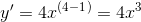The slope at every point of the functionhas a slope of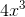.  To find the slope at the given point, substitute the x-value of the given point,, into the derivative function to find the slope.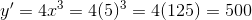### Example Question #1 : Algebra

Circle A is centered about the origin and has a radius of 5. What is the equation of the line that is tangent to Circle A at the point (–3,4)?

–3x + 4y = 1

3x – 4y = –25

3x + 4y = 7

3x – 4y = –1

3x – 4y = –25

Explanation:

The line must be perpendicular to the radius at the point (–3,4). The slope of the radius is given by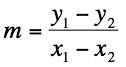The radius has endpoints (–3,4) and the center of the circle (0,0), so its slope is –4/3.

The slope of the tangent line must be perpendicular to the slope of the radius, so the slope of the line is ¾.

The equation of the line is y – 4 = (3/4)(x – (–3))

Rearranging gives us: 3x – 4y = -25

### Example Question #1 : How To Find The Equation Of A Tangent Line

Find the equation of a tangent line at point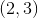if the function is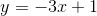.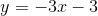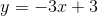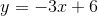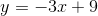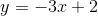Explanation:

To find the slope of the tangent line, it is necessary to determine the slope of the function.

The functionis already in the slope-intercept form,, and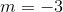.

Substitute the slope and the given pointinto the slope-intercept equation.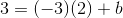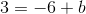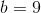Substitute the known slope and the y-intercept to the slope-intercept form.### All SSAT Upper Level Math Resources